# Beyond Mapping III

Topic 7: Linking Data Space and Geographic Space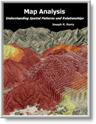Map Analysis book with companion CD-ROM for hands-on exercises

discusses the relationships among maps, map surfaces and data distributions
describes the direct link between numeric and geographic distributions
Normally Things Aren’t Normal  discusses the appropriateness of using traditional “normal” and percentile statistics

Explore Mapped Data describes creation of a Standardized Map Variable surface using Median and Quartile Range

Babies and Bath Water  discusses the information lost in aggregating field data and assigning typical values to polygons (desktop mapping)

establishes the concept of "data space" and how mapped data conforms to this fundamental view
discusses data clustering and its application in identifying spatial patterns

Note: The processing and figures discussed in this topic were derived using MapCalcTM software.  See www.innovativegis.com to download a free MapCalc Learner version with tutorial materials for classroom and self-learning map analysis concepts and procedures.

______________________________

Beware the Slippery Surfaces of GIS Modeling

(GeoWorld, May 1998, pg. 26)

GIS means different things to different people.  My favorite characterization is that “GIS technology is as different as it is similar to traditional mapping and data analysis.”  That statement leaves some room for both conventional and unconventional views of what GIS is, what it’s good for, and who uses it for what.

For example, traditional mapping focuses on points, lines and polygons as fundamental map features.  Spatial database management systems (desktop mapping packages) extend this view by linking the discrete “map objects” to descriptive information about them.  The nature of the maps, as well as the linkage, is as familiar as the map on the wall and the file cabinet beside it.  The paradigm also fits nicely into standard office software with only minimal education and training in unfamiliar spatial reasoning.

The problem is the traditional paradigm doesn’t fit a lot of reality.  Sure the lamppost, roadway and the parking lot at the mall are physical realities of a map’s set of points, lines and polygons.  Even very real (legally), though non-physical, property boundaries conceptually align with the traditional paradigm.  But not everything in space can be so discretely defined, nor is it as easily put in its place.

Obviously meteorological gradients, such as temperature and barometric pressure, don’t fit the P, L and P mold.  They represent phenomena that are constantly changing in geographical space, and are inaccurately portrayed as contour lines, regardless how precisely the lines are drawn.  By its very nature, a continuous spatial variable is corrupted by artificially imposing abrupt transitions.  The contour line is simply a mechanism to visually portray a 2-dimensional rendering of a 3-dimensional gradient.  It certainly isn’t a basic map feature, nor should it be a data structure for storage of spatially complex phenomena.

Full-featured GIS packages extend the basic P, L and P features to map surfaces that treat geographic space as a continuum.  The most familiar surface feature is the terrain you walk on, composed of hills and valleys and everything in between.  One of the unique characteristics of an elevation surface is well known to hikers—slope.  While hikers hate steep slopes, avalanches love them.  Another unique characteristic of surfaces determines the orientation for a portion of the surface—aspect.  Elk seek out southern exposures in the winter, but thirsty trees wouldn’t be caught dead there in a moisture-limited climate.

While a contour map of elevation lets your eye assess slope (closeness of lines) and aspect (downhill direction), it leaves the computer without a clue, as all it can see is a pile of numbers (digital data), not an organized set of lines (analog image).

Figure 1 links different views of surface data.  Keep in mind, that the computer doesn’t “see” any of them; they’re for human viewing enjoyment.  The traditional 2-D map view chooses a map value, then identifies all of the locations (mathematically implied) having that value.  That begs the traditional GIS 101 question, “Does a set of contours form point, line or area features?”  Actually, arguments can be made for any of the discrete feature types depending on the application.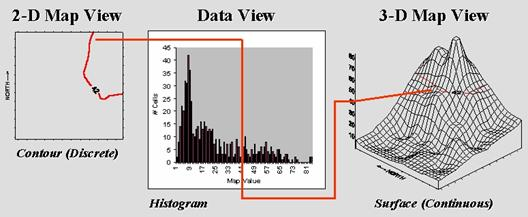Figure 1.  A histogram (numerical distribution) is linked to a map surface (geographic distribution) by a common axsis of map values.

However, it’s the discrete nature of a contour map and the irregular features spawned that restrict its ability to effectively represent continuous phenomena.  The data view uses a histogram to characterize a continuum of map values in “numeric” space.  It summarizes the number of times a given value occurs in a data set (relative frequency).

The 3-D map view forms a continuous surface by introducing a regular grid over an area.  The X and Y axes position the grid cells in geographic space, while the Z axis reports the numeric value at that location.  Note that the data and surface views share a common axis—map value.  It serves as the link between the two perspectives.  For example, the data view shows number of occurrences for the value 42 and the relative numerical frequency considering the occurrences for all other values.  Similarly, the surface view identifies all of the locations having a value of 42 and the relative geographic positioning considering the occurrences for all other values.

The concept of an “aggregation interval” is shared as well.  In constructing a histogram, a constant data step is used.  In the example, a data interval of 1.0 unit was used, and all fractional values were rounded, then “placed” in the appropriate “bin.”  In an analogous fashion, the aggregation interval for constructing a surface uses a constant geographic step, as well as a constant data step.  In the example, an interval of 1.0 hectare was used to establish the constant partitioning in geographic space.  In either case, the smaller the aggregation interval the better the representation.

Both perspectives characterize data dispersal.  The numeric distribution of data (a histogram’s shape of ups/downs) forms the cornerstone of traditional statistics and determines appropriate data analysis procedures.  In a similar fashion, a map surface establishes the geographic distribution of data (a surface’s shape of hills/valleys) and is the cornerstone of spatial statistics.  The assumptions, linkages, similarities and differences between these two perspectives of data distribution are the focus of the next few columns… we’ll dust off the old stat book together.

(GeoWorld, June 1998, pg. 28-30)

Some of the previous Beyond Mapping articles might have found you reaching for your old Stat 101 textbook.  Actually, the concepts used in mapped data analysis are quite simple—it’s the intimidating terminology and “picky, picky” theory that are hard.  The most basic concept involves a number line that is like a ruler with tic-marks for numbers from small to large.  However, the units aren’t always inches, but data units like number of animals or dollars of sales.  If you placed a dot for each data measurement (see top of figure 1), there would be a minimum value on the left (#animals = 0) extending to a maximum value on the right.  The rest of the points would fall on top of each other throughout the data range.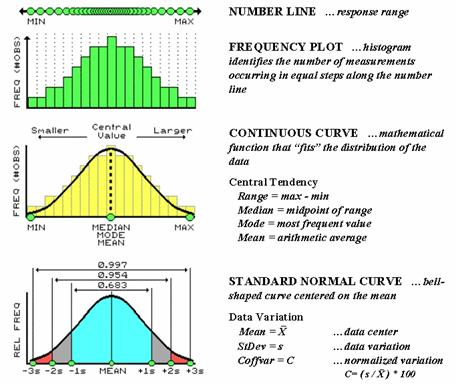Figure 1.  The distribution of measurements in “data space” is described by its histogram and summarized by descriptive statistics.

To visualize these data, we can look at the number line from the side and note where the measurements tend to pile up.  If the number line is divided into equally spaced “shoots” (like in pinball machine) the measurements will pile up to form a histogram plot of the data’s distribution.  Now you can easily see that most of the measurements fell about midrange.

In statistics, several terms are used to describe this plot and its “central tendency.”  The median identifies the “midway” value with half of the distribution below it and half above it, while the mode identifies the most frequently occurring value in the data set.  The mean, or average, is a bit trickier as it requires calculation.  The total of all the measurements is calculated and then divided by the number of measurements in the data set.

Although the arithmetic is easy (for a tireless computer), its implications are theoretically deep. When you calculate the mean and its standard deviation you’re actually imposing the “standard normal curve” assumption onto the histogram.  The bell-shaped curve is symmetrical with the mean at its center.  For the “normally distributed” data shown in the figure, the fit is perfect with exactly half of the data on either side.  Also note that the mean, mode and median occur at the same value for this idealized distribution of data.

Now let’s turn our attention to the tough stuff—characterizing the data variation about the mean.  When considering variation one must confront the concept of a standard deviation (StDev).  The standard deviation describes the dispersion, or spread, of the data around the mean.  It’s a consistent measure of the variation, as one standard deviation on either side of the mean “captures” slightly more than two-thirds of the data (.683 of the total area under the curve to be exact).  Approximately 95% of all the measurements are included within two standard deviations, and more than 99% are covered by three.

The larger the standard deviation, the more variable is the data, indicating that the mean isn’t very typical.  In GIS applications, a small standard deviation tells you there isn’t much variation in an area of interest.  However, a large standard deviation indicates a lot of variability and using a simple average to characterize the area is likely misleading.

So what determines whether a standard deviation is large or small?  That’s the role of the coefficient of variation (Coffvar).  This semantically-challenging mouthful simply “normalizes” the variation in the data by expressing the standard deviation as a percent of the mean—if it’s large, say over 50%, then there is a lot of variation and the mean is a poor estimator of what’s happening in a mapped area.  Keep this in mind the next time you assign an average value to map features, such as the average tree diameter for each forest parcel, or the average home value for each county.

A large portion of the variation can be “explained” through its spatial distribution.  Figure 2 shows a technique that brings statistics down to earth by mapping the standard normal curve in geographic space.  The procedure first calculates the mean and standard deviation for the typical response in a data set.  The data is then spatially interpolated into a continuous geographic distribution.  A standard normal variable surface is derived by subtracting the mean from the map value at each location (deviation from the typical), then dividing by the standard deviation (normalizing to the typical variation) and multiplying by a hundred to form a percent.  The result is that every map location gets a number indicating exactly how “typical” it is.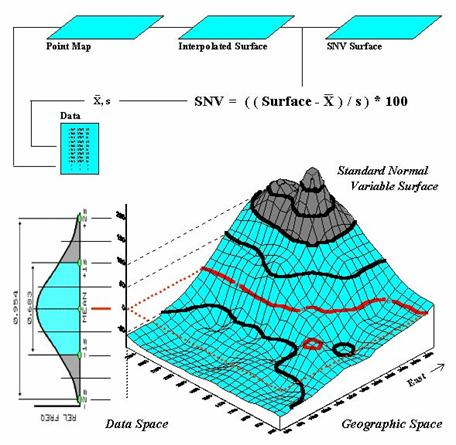Figure 2.  A “standard normal surface” identifies how typical every location is for an area of interest.

The contour lines draped on the SNV surface in the figure show half-StDev steps. Locations that are exactly the same as the mean equate to zero (typical areas).  The high peak in the northeast portion locates an area of unusually high response (>200% of a standard deviation above the mean).  The entire western portion of the map is characterized by responses below the typical, however there are no unusually low areas in this case.  The geographic distribution balances at zero (just as many locations above the mean as below), but the data distribution is not balanced (no unusually low responses).  This condition (asymmetrical data) muddles the standard normal procedure, but that discussion is reserved for techy-types via www.innovativegis.com/basis (select Column Supplements).

Normally Things Aren’t Normal

(GeoWorld, September 2007, pg. 18)

No matter how hard you have tried to avoid the quantitative “dark” side of GIS, you likely have assigned the average (Mean)  to a set of data while merrily mapping it.  It might have been the average account value for a sales territory, or the average visitor days for a park area, or the average parts per million of phosphorous in a farmer’s field.  You might have even calculated the Standard Deviation to get an idea of how typical the average truly was.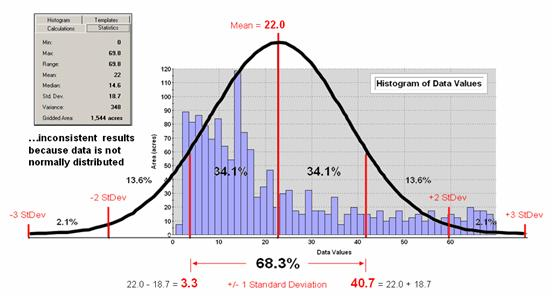Figure 1. Characterizing data distribution as +/- 1 Standard Deviation from the Mean.

But there is a major assumption every time you map the average—that the data is Normally Distributed.  That means its histogram approximates the bell curve shape you dreaded during grading of your high school assignments.  Figure 1 depicts a standard normal curve applied to a set of spatially interpolated animal activity data.  Notice that the fit is not too good as the data distribution is asymmetrical—a skewed condition typical of most data that I have encountered in over 30 years of playing with maps as numbers.  Rarely are mapped data normally distributed, yet most map analysis simply sallies forth assuming that it is.

A key point is that the vertical axis of the histogram for spatial data indicates geographic area covered by each increasing response step along the horizontal axis.  If you sum all of the piecemeal slices of the data it will equal the total area contained in the geographic extent of a project area.  The assumption that the areal extent is symmetrically distributed in a declining fashion around the midpoint of the data range hardly ever occurs.  The norm is ill-fitting curves with infeasible “tails” hanging outside the data range like the baggy pants of the teenagers at the mall.

As “normal” statistical analysis is applied to multiple skewed data sets (the spatial data norm) comparative consistency is lost.  While the area under the standard normal curve conforms to statistical theory, the corresponding geographic area varies widely from one map to another.

Figure 2 depicts an alternative technique involving percentiles.  The data is rank-ordered (either ascending or descending) and then divided into Quartiles with each step containing 25% of the data.  The Median identifies the breakpoint with half of the data below and half above.  Statistical theory suggests that the mean and median align for the ideal normal distribution.  In this case, the large disparity (22.0 versus 14.6) confirms that the data is far from normally distributed and skewed toward lower values since the median is less than the mean.  The bottom line is that the mean is over-estimating the true typical value in the data (central tendency).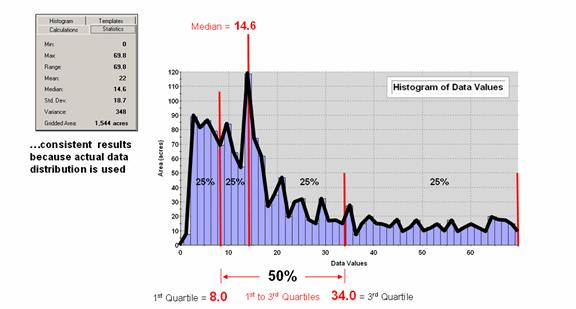Figure 2. Characterizing data distribution as +/- 1 Quartile from the Median.

Notice that the quartile breakpoints vary in width responding to the actual distribution of the data.  The interpretation of the median is similar to that of the mean in that it represents the central tendency of the data.  In an analogous manner, the 1st to 3rd quartile range is analogous to +/- 1 standard deviation in that it represents the typical data dispersion about the typical value.

What is different is that the actual data distribution is respected and the results always fit the data like a glove.  Figure 3 maps the unusually low (blue) and high (red) tails for both approaches—traditional statistics (a) and percentile statistics (b).  Notice in inset a) that the low tail is truncated as the fitted normal curve assumes that the data can go negative, which is an infeasible condition for most mapped data.  In fact most of the low tail is lost to the infeasible condition, effectively misrepresenting the spatial pattern of the unusually low areas.  The 2D discrete maps show the large discrepancy in the geographic patterns.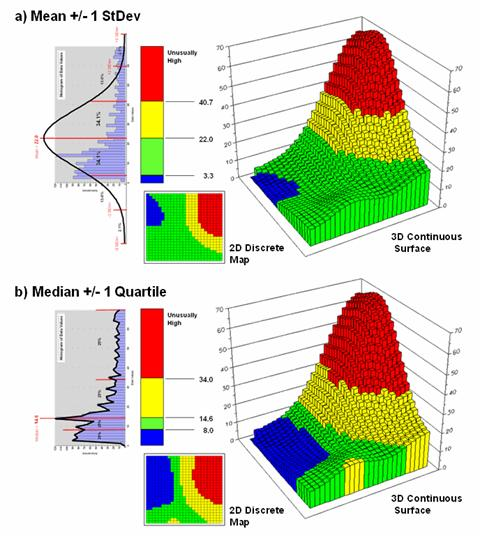Figure 3. Geographic patterns resulting from the two thematic mapping techniques.

The astute reader will recognize that the percentile statistical approach is the same as the “Equal Counts” display technique used in thematic mapping.  The percentile steps could even be adjusted to match the + /- 34.1, 13.6 and 2.1% groupings used in normal statistics.  Discussion in the next section builds on this idea to generate a standard variable map surface that identifies just how typical each map location is—based on the actual data distribution, not an ill-fitted standard normal curve …pure heresy.

(GeoWorld, October, 2007)

The previous section investigated the wisdom of using the arithmetic Average and Standard Deviation of a set of mapped data to represent its “typical” value and presumed variation.  The bottom line was that the assumptions ingrained in the calculations of an Average are rarely met for most map variables.  Their distributions are often skewed and seldom form an idealized bell-shaped curve.  In addition force-fitting a standard normal curve often extends the “tails” of the distribution into infeasible conditions, such as negative values.

The discussion suggested an alternative statistic, the Median, as a much more stable central tendency measure.  It is identifies the break point where half of the data is below and half is above ...analogous to the Average.  A measure of data variation is formed by identifying the Quartile Range from the lowest 25% of the data (1st quartile) and the uppermost 25% (4th quartile) …analogous to the Standard Deviation.  The approach consistently recognizes the actual balance point for mapped data and never force-fits a solution outside of the actual data range.

Figure 1.  Spatial Interpolation is used to generate the spatial distribution (continuous surface) inherent in a set of field data (discrete points).

This section takes the discussion a bit further by generating a Standardized Map Variable surface that identifies just how typical each map location is based on the actual data distribution, not an ill-fitted standard normal curve.  Figure 1 depicts the first step of the process involving the conversion of the discrete point data into its implied spatial distribution.  Notice that the relatively high sample values in the NE form a peak in the surface, while the low values form a valley in the NW.

Both the Average and Median are shown in the surface plot on the right side of the figure.  As discussed in the last section, the Average tends to over-estimate the typical value (central tendency) because the symmetric assumption of the standard normal curve “slops” over into infeasible negative values.  This condition is graphically reinforced in the figure by noting the lack of spatial balance between the area above and below the Average.  The Median, on the other hand, balances just as much of the project area above the Median as below.

Figure 2 extends this relationship by generating a Standardized Map Variable surface.  The calculation normalizes the difference between the interpolated value at each location and the Median using the equation shown in the figure (where Q_Range is the Quartile Range from the 2nd through the 3rd quartile).  Recall that each quartile represents 25% of the data beginning from the minimum value through the maximum value.  The result is that the Quartile Range captures the middle 50% of the data and represents the typical dispersion in the data.  The 1st and 4th quartiles represent unusually low and high values in the “tails” of the numerical distribution of the data.  The Standardized Map Variable plot shows you where these areas occur in the geographical distribution of the data—blue tones increasing low and red tones increasingly high.

Figure 2.  A Standardized Map Variable (SMV) uses the Median and Quartile Range to generate a statistically consistent rendering of the spatial distribution of a data set.

The real value of viewing your field collected data as a Standardized Map Variable (SMV) is that is consistent for all data.  You have probably heard that you can’t compare “apples and oranges” but with a SMV surface you can.  Figure 3 shows the results for two different variables for the same project area.

Figure 3.  Mapping the spatial distribution of field data enables discovery of important geographic patterns that are lost when the average is assigned to entire spatial objects.

SMV normalization enables direct comparison as percentages of the typical data dispersion within data sets and without cartographic confusion and inconsistency.  A dark red area is just as unusually high in variable 1 as it is in variable 2, regardless of their respective measurement units, numerical distribution or spatial distribution.

That means you can get a consistent “statistical picture” of the relative spatial distributions (where the low, typical and high values occur) among any mapped data sets you might want explore.  How the blue and red color gradients align (or don’t align) provides considerable insight into common spatial relationships and patterns of mapped data.

(GeoWorld, November, 2007)

The last two sections first challenged the appropriateness of  the ubiquitous assumption that all spatial data is Normally Distributed, and then suggested an alternative approach involving a Standardized Map Variable surface based on the “median and quartile range” instead of the usual “mean and standard deviation” …traditional statistics heresy, but most appropriate for spatial statistics.

This section takes the discussion to new heights (or is it lows?) by challenging the use of any scalar central tendency statistic to represent mapped data.  Whether the average or the median is used, a robust set of field data is reduced to a single value assumed to be same everywhere throughout a parcel.  This supposition is the basis for most desktop mapping applications that takes a set of spatially collected data (parts per million, number of purchases, disease occurrences, crime incidence, etc.), reduces all of the data to a single value (total, average, median, etc.) and then “paints” a fixed set of polygons with vibrant colors reflecting the scalar statistic of the field data falling within each polygon.

For example, the left side of figure 1 depicts the position and relative values of some field collected data; the right side shows the derived spatial distribution of the data for an individual reporting parcel.  The average of the mapped data is shown as a superimposed plane “floating at average height of 22.0” and assumed the same everywhere within the polygon.  But the data values themselves, as well as the derived spatial distribution, suggest that higher values occur in the northeast and lower values in the western portion.

Figure 1.  The average of a set of interpolated mapped data forms a uniform spatial distribution (horizontal plane) in continuous 3D geographic space.

The first thing to notice in figure 1 is that the average is hardly anywhere, forming just a thin band cutting across the parcel.  Most of the mapped data is well above or below the average.  That’s what the standard deviation attempts to tell you—just how typical the computed typical value really is.  If the dispersion statistic is relatively large, then the computed typical isn’t typical at all.  However, most desktop mapping applications ignore data dispersion and simply “paint” a color corresponding to the average regardless of numerical or spatial data patterns within a parcel.

Figure 2 shows how this can get you into a lot of trouble.  Assume the data is mapping an extremely toxic chemical in the soil that, at high levels, poses a serious health risk for children.  The mean values for both the West (22.0) and the East (28.2) reporting parcels are well under the “critical limit” of 50.0.  Desktop mapping would paint both parcels a comfortable green tone, as their typical values are well below concern.  Even if anyone checked, the upper-tails of the standard deviations don’t exceed the limit (22.0 + 18.7= 40.7 and 28.2 + 19.8= 48.0).  So from a non-spatial perspective, everything seems just fine and the children aren’t in peril.

Figure 2.  Spatial distributions and superimposed average planes for two adjacent parcels.

Figure 3, however, portrays a radically different story.  The West and East map surfaces are sliced at the critical limit to identify areas that are above the critical limit (red tones).  The high regions, when combined, represent nearly 15% of the project area and likely extend into other adjacent parcels.  The aggregated, non-spatial treatment of the spatial data fails to uncover the pattern by assuming the average value was the same everywhere within a parcel.

Figure 3.  Mapping the spatial distribution of field data enables discovery of important geographic patterns that are lost when the average is assigned to entire spatial objects.

Our paper mapping legacy leads us to believe that the world is composed of a finite set of discrete spatial objects—county boundaries, administrative districts, sales territories, vegetation parcels, ownership plots and the like.  All we have to do is color them with data summaries.  Yet in reality, few of these groupings provide parceling that reflects inviolate spatial patterns that are consistent over space and time with every map variable.  In a sense, a large number of GIS applications “throw the baby (spatial distribution) out with the bath water (data)” by reducing detailed and expensive field data to a single, maybe or maybe not, typical value.

(GeoWorld, July 1998, pg. 28)

Earlier discussion described a histogram plot and the old bell curve as a computer’s view of an individual map.  Now let’s extend those concepts to how a computer “visualizes” several maps at a time.  Fundamental to this perspective is recognition that digital maps are “numbers first, pictures later.”  In a computer, each map is a long list of numbers, with each value identifying a characteristic or condition at a particular location.

Following my usual recipe for journalistic suicide let’s consider the fundamental concepts of the dismal discipline of spatial statistics within the context of production agriculture.  For example, a map of phosphorous in the top layer of soil (0-5cm) in a farmer’s field contains values ranging from 22 to 140 parts per million.  The spatial pattern of these data is characterized by the relative positioning of the data values within a reference grid of cell locations.  The set of cells forming the analysis grid identifies the spatial domain (geographic space), while the map values identifies the data domain (data space).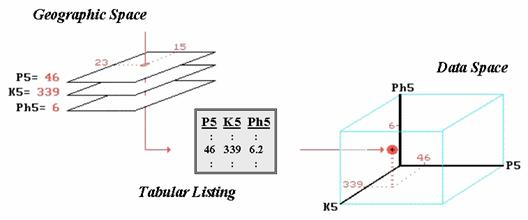Figure 1.  The map values for a series of maps can be simultaneously plotted in data space.

A dull and tedious tabular listing, as shown in the center of Figure 1, is the traditional human perspective of such data.  We can’t consume a long list of numbers, so we immediately turn the entire column of data into a single “typical” value (average) and use it to make a decision.  For the soil phosphorous data set, the average is 48.  A location in the center of the field (column 15, row 23 of the analysis grid) has a phosphorous level of 46 that is close to the average value (in a data sense, not a geographic sense).  But recall that the data range tells us that somewhere in the field there is at least one location that is less than half (22) and another that is nearly three times the average (140), so the average value doesn’t tell it all.

Now consider additional map surfaces of potassium levels (K5) and soil acidity (Ph5), as well as phosphorous (P5), for the field.  As humans we could “see” the coincidence of these data sets by aligning their long columns of numbers in a spreadsheet or database.  Specific levels for all three of the soil measurements at any location in the field are identified as rows in the table.  However, the combined set of data is even more indigestible, with the only “humane” view of map coincidence being the assumption that the averages are everywhere— 48, 419, 6.2 in this example.  The center location’s data pattern of 46, 339, and 6.0 is fairly similar to the pattern of the field averages, but exactly how similar?  Which map locations in the field are radically different?

Before we can answer these questions, we need to understand how the computer “sees” similarities and differences in multiple sets of data.  It begins with a three-dimensional plot in data space as shown on the right side of figure 1.  The data values along a row of the tabular listing are plotted resulting in each map location being positioned in the imaginary box based on its data pattern.  Similarity among the field’s soil response patterns are determined by their relative positioning in the box— locations that plot to the same position in the data space box are identical; those that plot farther away from one another are less similar.

How the computer “measures” the relative distance becomes the secret ingredient in assessing data similarity.  Actually it’s quite simple if you revisit a bit of high school geometry, but I bet you thought you had escaped all that awful academic fluff when you entered the colorful, fine arts world of computer mapping and geo-query.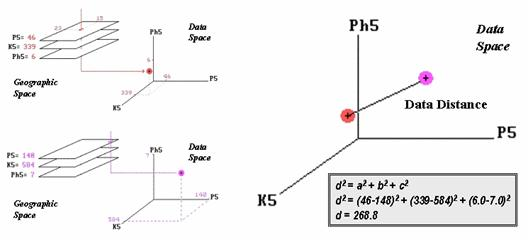Figure 2.  Similarity is determined by the data distance between two locations and is calculated by expanding the Pythagorean Theorem.

The left side of figure 2 shows the data space plots for soil conditions at two locations in the farmer’s field.  The right side of the figure shows a straight line connecting the data points whose length identifies the data distance between the points.  Now for the secret— it’s the old Pythagorean theorem of c2 = a2 + b2 (I bet you remember it).  However, in this case it looks like d2 = a2 + b2 + c2 as it has to be expanded to three dimensions to accommodate the three maps of phosphorous, potassium and acidity (P5, K5 and Ph5 axes in the figure).  All that the Wizard of Oz (a.k.a., computer programmer) has to do is subtract the values for each condition between two locations and plug them into the equation.  If there are more than three maps, the equation simply keeps expanding into hyper-data space which as humans we can no longer plot or easily conceptualize.

The underlying principle is that the smaller the data distance, the greater the similarity between locations.  That’s the basics, but the subtle nuances, such as normalizing the axes, provide fodder for next month’s discussion of mapping similarity and clustering data into similar spatial patterns— a useful tool down on the farm, but just as useful for retailers, land planners, foresters, real estate agents and just about any GIS user.

Identify Data Patterns

(GeoWorld, August 1998, pg. 26-27)

The previous section introduced the concept of data distance.  While most of us are comfortable with the concept of distance in geographic space, things get a bit abstract when we move from feet and meters in the real world to data units in data space.

Recall that data space is formed by the intersection of two or more axes in a typical graph.  If you measured the weight and height of several students in your old geometry class, each of the paired measurements for a person would plot as a dot in XY data space locating their particular weight (X axis) and height (Y axis) combination.  A plot of all the data looks like a shotgun blast and is termed a scatter plot.

The scatter plots for a lot of data sets form clusters of similar measurements.  For example, two distinct groups might be detected in the geometry class’s data—the demure cheerleaders (low weight and height) and the football studs (high weight and height).  Traditional (non-spatial) data analysis stops at identifying the groupings.  Spatial statistics, however, extends the analysis to geographic contexts.

If seating “coordinates” accompanied the classroom data you might detect that most of the cheerleaders were located in one part of the room, while the studs were predominantly in another.  Further analysis might show a spatial relation between the positioning of the groups and proximity of the teacher—cheerleaders in front and studs in back.

The linking of traditional statistics with spatial analysis capabilities (such as proximity measurement) provides insight into the spatial context and relationships inherent in the data.  The only prerequisite is “tagging” geographic coordinates to the measurements.  Until recently, this requirement presented quite a challenge and spatial coordinates were rarely included in most data sets.  With the advent of GPS and Geo-Coding based on street address, geographic tagging has become a whole lot easier.

The new hurdle, however, isn’t so much technical as it is social.  Most data analysis types aren’t familiar with spatial concepts, while most GIS types aren’t familiar with data mining and knowledge discovery procedures.  With GIS moving beyond mapping and geo-query, understanding data analysis concepts becomes as important as understanding geographic concepts.  With this in mind, let’s see one way a computer might identify numerical patterns within a set data.

The scatter plot in inset (a) of figure 1 shows weight/height data that might have been collected in your old geometry class.  Note that all of the students do not have the same weight/height measurements and that many vary widely from the class average.  Your eye easily detects two groups (low/low and high/high) in the plot but the computer just sees a bunch of numbers.  So how does it identify the groups without seeing the scatter plot?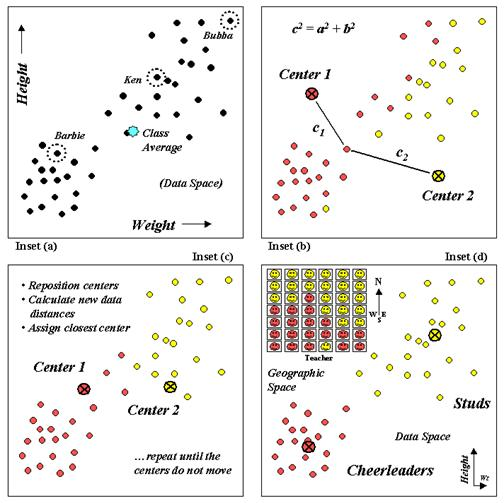Figure 1.  Clustering uses repeated data distance calculations to identify numerical patterns in a data set.

One approach (termed k-means clustering) arbitrarily establishes two cluster centers in the data space (inset (b)).  The data distance to each weight/height measurement pair is calculated and the point is assigned to the closest cluster center.  Recall from last month’s article that the Pythagorean theorem of c2 = a2 + b2 is used to calculate the data distance and can be extended to more than just two variables (hyper-data space).  It should be at least some comfort to note that the geometry you learned in high school holds for the surreal world of data space, as well as the one you walk on.  In the example, c1 is smaller than c2 therefore that student’s measurement pair is assigned to cluster center 1.   The remaining student assignments are identified in the scatter plot by their color codes.

The next step calculates the average weigh/height of the assigned students and uses these coordinates to reposition the cluster centers (inset (c)).  Other rounds of data distances, cluster assignments and repositioning are made until the cluster membership does not change (i.e., the centers do not move).

Inset (d) shows the final groupings with the big folks (high/high) differentiated from the smaller folks (low/low).  By passing these results to a GIS the data space pattern (clumps of similar measurements) can be investigated for geographic space patterns.

The positioning of these data in the real world classroom (upper left portion of inset (d)) shows a distinct spatial pattern between the two groups—smaller folks in front and bigger folks in the rear.  Like before, you simply see these things but the computer has to derive the relationships (distance to similar neighbor) from a pile of numbers.

What is important to note is that analysis in data space and geographic space has a lot in common.  In the example, both “spaces” are represented as XY coordinates—weight/height measurements in data space (characteristics) and longitude/latitude in geographic space (positioning).  Data distance is used to partition the measurements into separate groups (data pattern).  Geographic distance is used to partition the locations into separate groups (spatial pattern).  In both instances the old Pythagorean Theorem served as the procedure for measuring distance.

So who cares?  We have gotten along for years with data analysts and mapmakers doing their own thing.  Maps are maps and data are data, right?  Not exactly, at least not any more… with the advent of the digital map, maps are data (not pictures).  Information on the relative positioning and coincidence among mapped variables extend traditional data analysis.  Likewise, the digital nature of maps provides data analysis tools that enable us to “see” geographic space in abstract terms (decision-making) beyond traditional descriptions of precise placement of physical features (inventory).